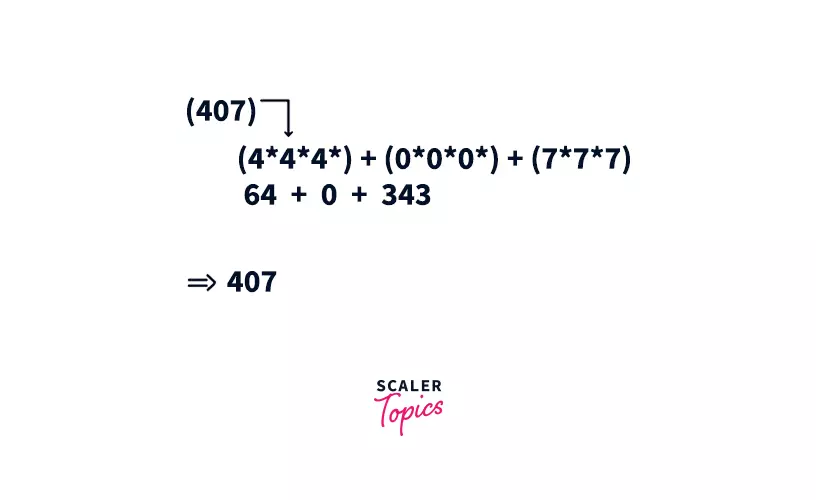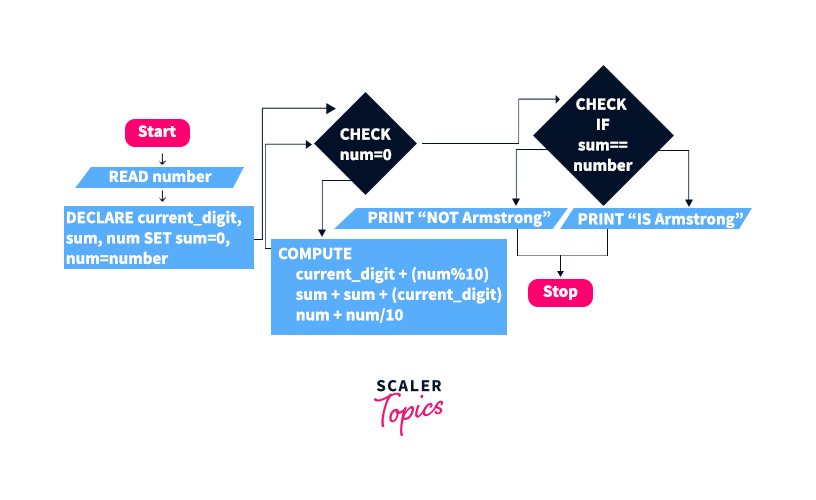Certificate

### Learn on Scaler Topics and get certified.# Armstrong Number in C

Learn about What is Armstrong Number in C along with example program.Challenge Inside! : Find out where you stand! Try quiz, solve problems & win rewards!

## Overview

In numerical number theory, the Armstrong number definition is the number in any given number base, which forms the total of the same number, when each of its digits is raised to the power of the number of digits in the number.

## Scope

• In this article we will understand what is an Armstrong Number.
• We will understand the algorithm to check if a number is Armstrong or not along with its code.

## What is an Armstrong Number?

A number is thought of as an Armstrong number if the sum of its own digits raised to the power number of digits gives the number itself. For example, 0, 1, 153, 370, 371, 407 are three-digit Armstrong numbers and, 1634, 8208, 9474 are four-digit Armstrong numbers and there are many more.

Let’s consider 407 since it is a three-digit number so it could be expressed as:Since 407 can be expressed as a sum of the cube of its digits, hence it is an Armstrong number.

We will now see an Algorithm to check for the number whether it’s an Armstrong number or not. So following are the steps that we would need to follow to test for the Armstrong number. The idea is to read one digit at a time from the back of the number, if the number is 4 0 7, then we will operate on 7 then 0, and then 4.

## Algorithm (for a 3-digit Number)

Step 1: Start.

Step 2: Read an integer input number.

Step 3: Declare andInitialise the variables current_digit , sum = 0 and num = number.

Step 4: Repeat Steps 5 to 7 Until num > 0.

Step 5: current_digit = (num % 10).

%10 return the last digit of the current number.

Step 6: sum = sum + (current_digit * current_digit * current_digit).

The variable current_digit is multiplied three times because we are checking for a three-digit Armstrong number.

Step 7: num = num / 10

After processing the last digit we need to remove it. /10 will give an integer such that the order of the number will be reduced and the next digit will become the last digit.

Step 8: Check if sum == number. Then Print “It is an Armstrong Number.” Else Print “It is not an Armstrong Number.”

Step 9: END

## Flowchart## Three-Digit Armstrong Number Example Code in C

Let’s take a three-digit number 371 and below is the C program that tells whether it is an Armstrong number or not.

#include<stdio.h>
#include<math.h>

int isArmstrong(int number) {
int current_digit, sum = 0, num = number, number_of_digits;

while (num > 0) {
current_digit = num % 10;
// Calculating the power of the remainder using pow() and storing in sum.
sum = sum + pow(current_digit, 3);
num = num / 10;
}

// Return 1 if the number is Armstrong else return 0.
if (sum == number) {
return 1;
} else {
return 0;
}
}

int main() {
int number = 371, isArmstrongNumber;

if (sum == number){
printf("%d is an Armstrong Number.", number);
} else {
printf("%d is not an Armstrong Number.", number);
}

// Calling the isArmstrong function.
isArmstrongNumber = isArmstrong(number);

if (isArmstrongNumber == 1) {
printf("%d is an Armstrong Number.", number);
} else {
printf("%d is not an Armstrong Number.", number);
}

return 0;
}


### Output:

371 is an Armstrong Number.


## Algorithm (for N-digit Number)

Step 1: Start

Step 2: Read an integer input number.

Step 3: Declare andInitialise the variables current_digit, sum = 0, digits = 0 and num = number.

Step 4: Calculate the number of digits in the input integer number and store it in the variable number_of_digits.

Step 5: Repeat Steps 5 to 7 Until num > 0

Step 6: current_digit = (num % 10)

Step 7: sum = sum + mnumber_of_digits

Step 8: num = num / 10

Step 9: Check if sum == number Then

Print “It is an Armstrong Number.”

Else

Print “It is not an Armstrong Number.”

Step 10: END

## Armstrong Number Code for N Digit Number in C Using Functions

Now In this C program, we will use different functions for checking for the Armstrong number. The output of this will be the same as the program that we have discussed above, but the structure of the program is changed. It is generally a good practice to use functions while making a program. This makes the code readable and helps in easy debugging.

### Code:

#include <stdio.h>
#include <math.h>

int countNumberOfDigits(int number) {
// Calculating the number of digits.
while (number > 0) {
// This will decrease the order of the number in each iteration.
number = number / 10;
number_of_digits++;
}

return number_of_digits;
}
int isArmstrong (int number) {
int current_digit, sum = 0, num = number, number_of_digits;
number_of_digits = countNumberOfDigits(num)
while (num > 0) {
current_digit = num % 10;
// Calculating the power of the remainder using pow() and storing in sum.
sum = sum + pow(current_digit, number_of_digits);
num = num / 10;
}
// return 1 if the number is Armstrong else return 0.
if (sum == number)
return 1;
else
return 0;
}

int main() {
int number, isArmstrongNumber;
scanf("%d", &number);
isArmstrongNumber = isArmstrong(number);
if(isArmstrongNumber == 1)
printf("%d is an Armstrong Number.", number);
else
printf("%d is not an Armstrong Number.", number);

return 0;
}


### Output:

Enter a Number: 54748
54748 is an Armstrong Number.


## Analysis of Algorithm

### Time Complexity

We are processing each digit of the number, therefore time complexity will be O(X), where X = log10(N) and N is the number we are checking for being an Armstrong number.

### Space Complexity

We are not using any extra space, therefore the time complexity will be $O(1)$.

## Conclusion

• We have discussed what are Armstrong numbers and then we have seen a C program to check for a three-digit number whether it is Armstrong or not.
• We have learned about a more generalized version of it for N-digit numbers.
Challenge Time!Time to test your skills and win rewards! Note: Rewards will be credited after the next product update.